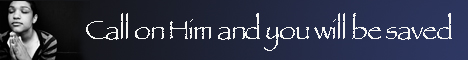Note:  Do not rely on this information. It is very old.

# Mensuration

Mensuration is that branch of applied mathematics which deals with the lengths of lines, areas of surfaces, and volumes of solids, however irregular may be their form. The length of a line is expressed by saying how many units (such as feet, chains, metres) it contains, and it is practically measured by applying the actual unit in the form of a rule or chain to it. The area of a surface is expressed in sums of some unit, such as a square foot, or an acre, but the unit itself does not require to be actually placed on the surface. It is sufficient to measure the lengths of certain lines, and from these the area can be calculated. If we are dealing with a rectangular figure, the area is given b\ multiplying the lengths of two adjacent sides. The area of a triangle is half the product of the base and height, and the area of any plane figure, such as a field, can be found by dividing it up into suitable triangles, finding their areas and adding the results, if the boundary of the field is curved, certain portions are taken which will approximate to parts of a circle, an ellipse, or other such curves, and calculating the areas by using the known formulae for those figures. The volumes of solids are found by the application of similar rules. All the rules and formula; used in mensuration can be proved by geometry, etc., but it is not necessary to know the proof in order to use the rules. Since these rules are few and simple, mensuration is an easy branch of practical science.